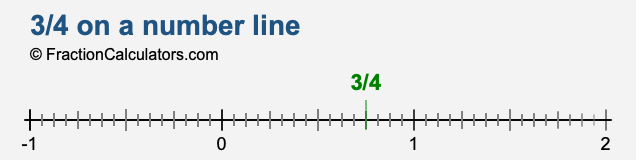3/4 on a number line

Here we will explain what a number line is and show you how to represent 3/4 on a number line. We find this helpful when dealing with fractions such as 3/4, because it shows how small or large 3/4 is in relation to other numbers or values.

First of all, a number line is a straight horizontal line marked with numbers in equal intervals. It increases as you go from left to right and decreases as you go from right to left. It has positive numbers to the right of 0 and negative numbers to the left of 0.

So where does 3/4 go on a number line? The fraction 3/4 is the same as 75 divided by 40, which is 0.75. Therefore, we simply find 0.75 on our number line, like this:Also, note that negative 3/4 would be the same distance from 0, but to the left side of 0 instead of the right side.

Fractions on a Number Line
Need us to put another fraction on a number line? No problem. Please submit your fraction below.

Represent / on a number line.

3/5 on a number line
Here is the next fraction on our list that we have represented on a number line for you.